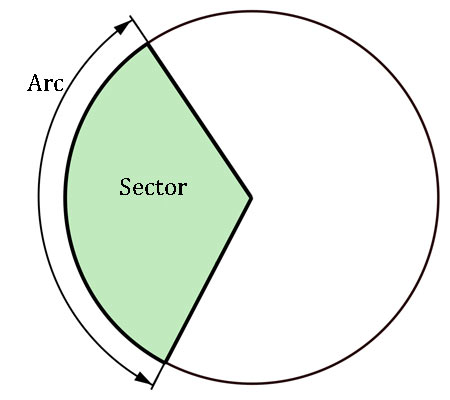A# arcAn arc is any part of a curved line or part of the circumference of a circle; the word comes from the Latin arcus for a bow, which also gives rise to "arch". Arc length is the distance along part of a curve.

For a circle of radius r, the length (s) of an arc covering θ degrees on that circle is given by

s = 2r × π × θ/360.

If the angle is measured in radians the length of the arc is given by the product of the radius and the angle, i.e., s = r θ. The arc is said to subtend the angle θ at the center of the circle.